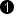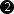## Operator ReSyncToLine

The operator ReSyncToLine is used to re-synchronize calculation results with the content of the current line. Thus, calculation results which are available at the end of a line can be applied to the same line. This allows the implementation of multiple-pass algorithms at the line level.

ReSyncToLine fetches the last pixel of each line on all k input links PI[k]. Also the current line at input link I is stored. After the last line pixel of the inputs is processed in the operator, the content of the line is output at the link O. The other output links PO0..PO63 output the fetched values of PI0..PI63. The PO values are held constant. All PO and O output ports are synchronous.

All input links (I and PI0..PI63) have to be fully synchronous, i.e. they have to be sourced by the same M-type operator through an arbitrary network of O type operators.

The operator delays the output by one line.

### I/O Properties

Property Value
Operator Type M
PI[k], data input
PO[k], data output

Bit Width [1, 64][1, 64]as I as PI[k]
Arithmetic {unsigned, signed} {unsigned, signed} as I as PI[k]
Parallelism any as I as I as I
Kernel Columns any any as I as PI[k]
Kernel Rows any any as I as PI[k]
Img Protocol {VALT_IMAGE2D, VALT_LINE1D} as I as I as I
Color Format any any as I as PI[k]
Color Flavor any any as I as PI[k]
Max. Img Width any as I as I as I
Max. Img Height any as I as I as I
 The range of the input bit width is [1, 64] for unsigned inputs. For signed inputs, the range is [2, 64]. For unsigned color inputs, the range is [3, 63] and for signed color, the range is [6, 63].

Synchronous and Asynchronous Inputs

• All inputs are synchronous to each other i.e. they have to be sourced by the same M-type operator through an arbitrary network of O-type operators.

None